# Confidence Intervals

Confidence intervals are an intuitive way to quantify the uncertainty in the observed metric deltas. A 95% confidence interval should contain the true effect 95% of the time. This means that if we ran an experiment 100 times, the true value of the metric delta should be inside the confidence intervals 95 times.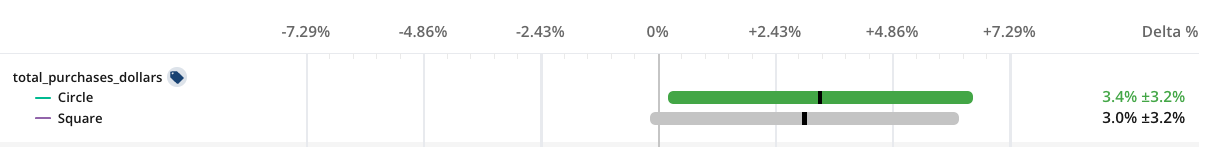In practical terms, a 95% confidence interval that doesn't contain zero (the green bar above) represents a statstically significant result (with α = 0.05). Only 5% of the time would we expect to see that confidence interval if the true effect was zero (a false positive). Larger confidence intervals imply less certainity in the exact size of the effect with a larger range of likley values.

## Computing Confidence Intervals​

Confidence intervals are calculated using a two-sample z-test. This requires knowledge of the variance in the metric delta we're measuring, which is derived differently depending on the type of metric (details here).

For the absolute metric delta, the confidence interval is given by: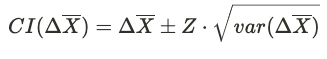where:

• Z is the z-statistic for the desired significance level (1.96 for two-sided 95% confidence interval)
• var(ΔX-bar) is the variance of the absolute delta

Similarly, the confidence interval for the relative metric delta is: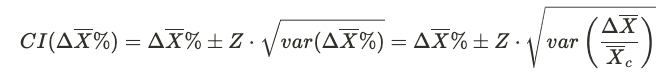Since the relative delta is a ratio of 2 variables, we must take care to properly account for the variance of both the numerator and denominator. Applying the delta method with the assumption of independent test and control groups yields: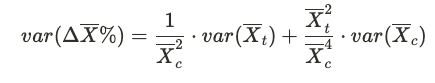## Welch's T-test for Small Sample Sizes​

For small sample sizes, we use Welch's t-test instead of a standard z-test. This statistical test is a better choice for handling samples of unequal size or variance without increasing the false positive rate. The structure of the confidence interval calculation remains the same as above, but instead of the z-statistic we use the t-statistic with degrees of freedom ν given by: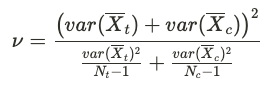Where Nt and Nc are the number of user in the test and control groups, respectively. Note that for large number of degrees of freedom, the t-statistic converges with the z-statistic. Therefore, Welch's t-test is used only when ν < 100.